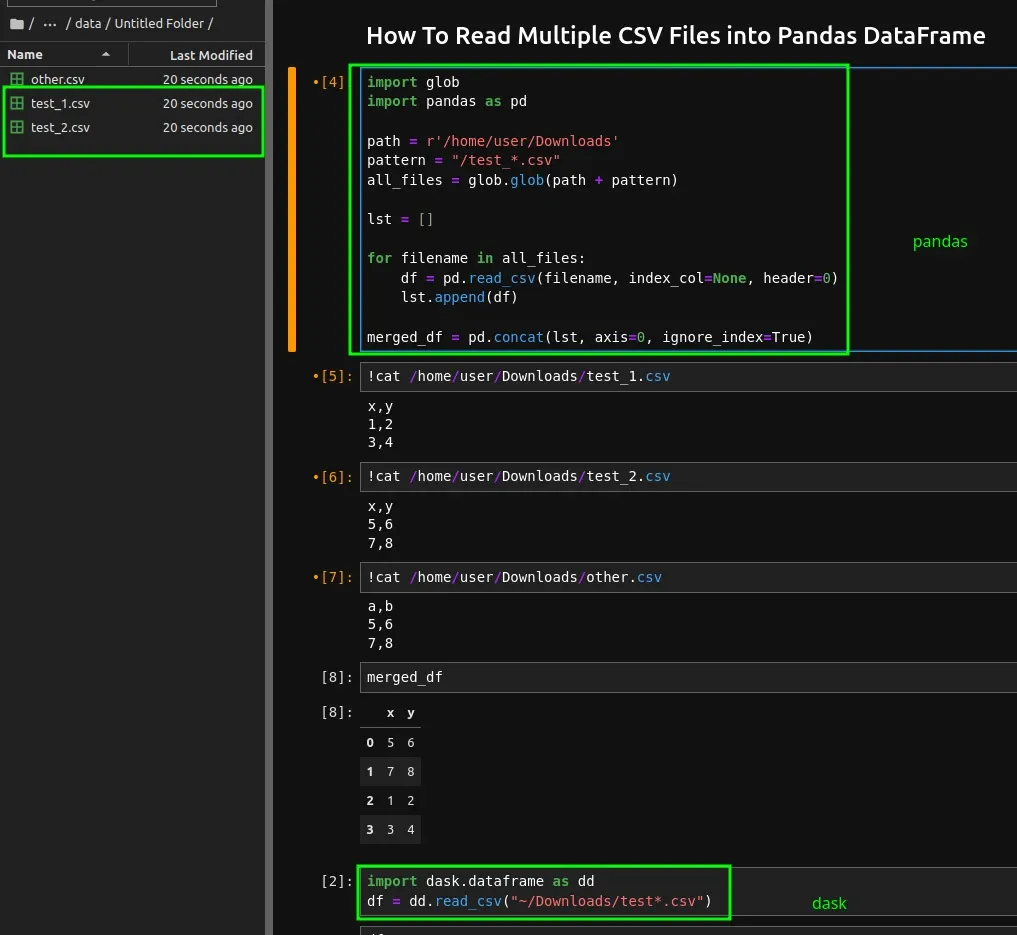To read multiple CSV file into single Pandas DataFrame we can use the following syntax:

(1) Pandas read multiple CSV files

``````path = r'/home/user/Downloads'
all_files = glob.glob(path + "/*.csv")

lst = []

for filename in all_files:
lst.append(df)

merged_df = pd.concat(lst, axis=0, ignore_index=True)
``````

``````import dask.dataframe as dd
``````

## Pandas Example

Suppose that we would like to read all CSV files:

• located in folder - `/home/user/Downloads`
• by pattern - `/test_*.csv` - starting with `test_` and ending on `.csv`

We can use the following code:

``````import glob
import pandas as pd

pattern = "/test_*.csv"
all_files = glob.glob(path + pattern)

lst = []

for filename in all_files:
lst.append(df)

merged_df = pd.concat(lst, axis=0, ignore_index=True)
``````

Let's say that we have the following files in this folder:

• other.csv
• test_1.csv
• test_2.csv

In the final DataFrame - merged_df we will have content only from files - test_1.csv and test_2.csv:As an alternative solution we can use the dask module to read multiple CSV files. To install Dask you can visit: dask or use: `pip install dask`.
``````import dask.dataframe as dd